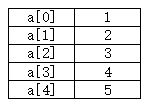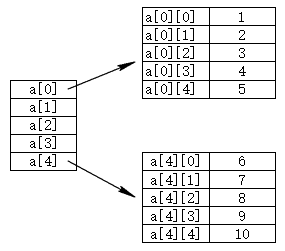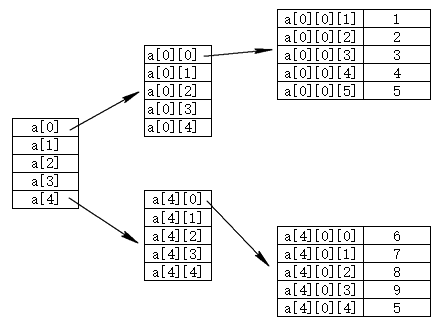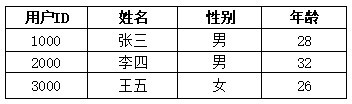• Java 教程
• Java 简介
• Java 基础
• Java 运算符
• Java 表达式
• Java 流程控制
• Java 数组
• Java 面向对象
• Java 集合类
• Java 异常处理
• Java 泛型
• Java 内置工具
• 参考手册

# 多维数组## 声明数组

### 一维数组

```<数据类型>[] <变量名>;

<数据类型> <变量名>[];```

```// 声明一个拥有10个元素的一维数组
int[] a = new int;```

### 二维数组

`<数组类型>[][] <变量名> = {{}, {}, ...};`

```// 合法
int[][] a = {
{1, 2, 3, 4, 5},
{6, 7, 8, 9, 10}
};```

`<数组类型>[][] <变量名>= new <数组类型>[<大小>][<大小>];`

```int[][] a = new int; // 合法
int[][] a = new int[]; // 合法，未定义 a 数组元素数组的长度
int[][] a = new int[]; // 非法
int[][] a = new int[][]; // 非法```

### 三维数组

```int[][][] a = {
{
{1, 2, 3},
{4, 5, 6}
},
{
{7, 8, 9},
{10, 11, 12}
}
};```

```int[][][] a = new int; // 合法
int[][][] a = new int[]; // 合法
int[][][] a = new int[][]; // 合法
int[][][] a = new int[][]; // 非法
int[][][] a = new int[][]; // 非法
int[][][] a = new int[]; // 非法```

## 多维数组示例```public class Demo {

public static void main(String[] args) {
// 声明一个能存放3个用户的数组
Object[][] users = new Object[];

// 初始化数组
users = new Object[]{1000, "张三", "男", 28};
users = new Object[]{2000, "李四", "男", 32};
users = new Object[]{3000, "王五", "女", 26};

// 迭代输出用户信息
for(Object[] user : users) {
System.out.printf("ID=%d\tNAME=%s\tSEX=%s\tAGE=%d\n", user);
}
}

}```

```ID=1000	NAME=张三	SEX=男	AGE=28
ID=2000	NAME=李四	SEX=男	AGE=32
ID=3000	NAME=王五	SEX=女	AGE=26```